## Per Unit System:

While carrying out the analysis of electrical machines (or electrical machine systems), it is usual to express voltage, current, VA and impedance in Per Unit System (or percentage) of the base or reference values of these quantities. The per unit (pu) value of any quantity is defined as the ratio of: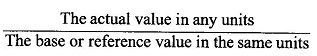While the base values can be selected arbitrarily, it is normal to choose the rated value of the device as its base values.

### Advantages of Per Unit System:

There are two important advantages that accrue from the use of the pu system. First, the parameters of transformers as well as rotating machines lie roughly in the same range of numerical values irrespective of their ratings if expressed in Per Unit System of their ratings; correctness of analysis becomes immediately obvious in this system.

Second, the pu system is most convenient in power systems as it relieves the analyst of the need to refer circuit quantities to one or other side of the transformers. It is a universal practice to use the pu system for modelling real-life large integrated power systems and in computer simulation of machine systems for their transient and dynamic analysis.

Base values of various quantities are related to each other by the usual electrical laws. For a single-phase system,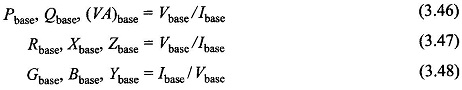Always, (VA)base and Vbase are first selected and their choice automatically fixes the other base values as per Eqs (3.46)-(3.48). It immediately follows from these equations that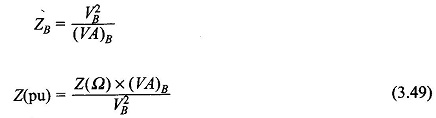In large devices and systems it is more practical to express the bases in kVA/ MVA and kV. Then Eq. (3.49) is written as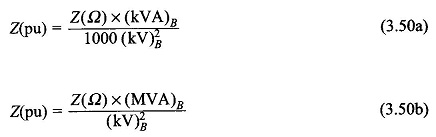When (MVA)B and (kV)B are modified, the new pu impedance is given by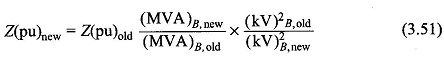It can be easily shown [7, pp 101-103] that in a transformer equivalent circuit using pu notations, the need for an ideal transformer is eliminated because the pu impedance of a transformer is the same whether computed from the primary or secondary side so long as the voltage bases on the two sides are selected in the ratio of transformation. A procedure universally adopted is to translate all quantities to pu values for carrying out analysis and to convert the results obtained back to actual units.

As has been mentioned earlier the pu parameters of transformers (and electric machines) lie within a narrow range. For example, the magnetizing current normally lies between 0.02 and 0.05 pu, the equivalent resistance between 0.005 pu (large transformers) and 0.02 pu (small transformers) and the equivalent reactance usually varies from 0.05 (large) to 0.1 pu (small transformers). This information helps a great deal in comparing units of a given size made by various manufacturers.

In the 3-phase system, the bases are chosen as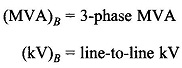Assuming star connection (equivalent star can always be found),which relationship is indeed the same as Eq. (3.50) for the single-phase system.

Consider three impedances, Z each, connected in delta. Then with 3-phase (MVA)B and line-to-line (kV)B,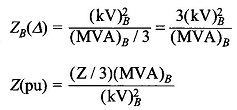Since Z/3 is the equivalent star impedance, the pu impedance for delta or its equivalent star is the same for a given 3-phase MVA base and line-to-line kV base.

Scroll to Top